# Explain about Message Authentication Code algorithm.?

In cryptography, a message authentication code, sometimes known as a tag, is a short piece of information used to authenticate a message—in other words, to confirm that the message came from the stated sender and has not been changed.

Basic block of Message Authentication Code (MAC)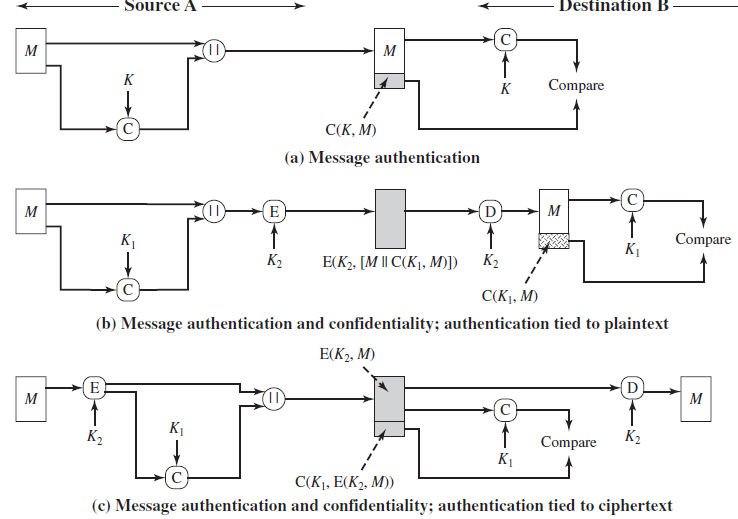• A secret key K is used to generate a small fixed-size block of data, known as a cryptographic checksum  or MAC, that is appended to the message.

MAC =  C(K , M )   where

C = MAC function

K = shared secret key

M = input message

It is assumed that only the receiver and the sender know the identity of the secret key, and if the received MAC matches the calculated MAC, then

1. The receiver is assured that the message has not been altered.
• If an attacker alters the message but does not alter the MAC, then the receiver’s calculation of the MAC will differ from the received MAC. Because the attacker is assumed not to know the secret key, the attacker cannot alter the MAC to correspond to the alterations in the message.
2.  The receiver is assured that the message is from the alleged sender.
• Because no one else knows the secret key, no one else could prepare a message with a proper MAC.
3. If the message includes a sequence number (such as is used with HDLC, and TCP), then the receiver can be assured of the proper sequence because an attacker cannot successfully alter the sequence number.
• A MAC function is similar to encryption. One difference is that the MAC algorithm need not be reversible, as it must be for decryption.
• In general, the MAC function is a many-to-one function. The domain of the function consists of messages of some arbitrary length, whereas the range consists of all possible MACs and all possible keys.
•  If an n -bit MAC is used, then there are 2n  possible MACs, whereas there are N  possible messages with N >>  2n . Furthermore, with a k -bit key, there are 2k  possible keys.
• Typically, it is preferable to tie the authentication directly to the plaintext, so the method of Figure b is used.

Security Requirements for MACs

• In assessing the security of a MAC function, we need to consider the types of attacks that may be mounted against it. Hence it needs to satisfy the listed requirements.
1. The first requirement deals with message replacement attacks, in which an opponent is able to construct a new message to match a given MAC, even though the opponent does not know and does not learn the key.
2. The second requirement deals with the need to thwart a brute-force attack based on chosen plaintext.
3. The final requirement dictates that the authentication algorithm should not be weaker with respect to certain parts or bits of the message than others.

Brute-Force Attack

• Requires known message-tag pairs
• A brute-force method of finding a collision is to pick a random bit string y and check if H(y) = H(x)
• Two lines of Attack
• Attack the key space
• If an attacker can determine the MAC key then it is possible to generate a valid MAC value for any input x
• Attack the MAC value
• Objective is to generate a valid tag for a given message or to find a message that matches a given tag

Cryptanalysis

• Cryptanalytic attacks seek to exploit some property of the algorithm to perform some attack other than an exhaustive search.
• An ideal MAC algorithm will require a cryptanalytic effort greater than or equal to the brute-force effort.
• There is much more variety in the structure of MACs than in hash functions, so it is difficult to generalize about the cryptanalysis of MACs

Implementation of MAC algorithms

• In essence, the security of MAC depends on the security of the underlying algorithm.
• There is much more variety in the structure of MACs than in hash functions, so it is difficult to generalize about the cryptanalysis of MACs.

We will consider two types of MACs developed:

1. MAC Based on Hash Functions: HMAC
2. Cipher based MAC: DAA and CMAC

1. MACs Based on Hash Functions: HMAC

• There has been increased interest in developing a MAC derived from a cryptographic hash function
• Motivations:
• Cryptographic hash functions such as MD5 and SHA generally execute faster in software than symmetric block ciphers such as DES.
• Library code for cryptographic hash functions is widely available.
• HMAC has been chosen as the mandatory-to-implement MAC for IP security, and is used in other Internet protocols, such as SSL.

HMAC Design Objectives

RFC 2104 lists the following objectives for HMAC:

1. To use, without modifications, available hash functions. In particular, hash functions that perform well in software, and for which code is freely and widely available.
2. To allow for easy replaceability of the embedded hash function in case faster or more secure hash functions are found or required.
3. To preserve the original performance of the hash function without incurring a significant degradation.
4. To use and handle keys in a simple way.
5. To have a well understood cryptographic analysis of the strength of the authentication mechanism based on reasonable assumptions about the embedded hash function.

RFC 2104 lists the following design objectives for HMAC:

• To use, without modifications, available hash functions. In particular, hash functions that perform well in software, and for which code is freely and widely available.

• To allow for easy replaceability of the embedded hash function in case faster or more secure hash functions are found or required.

• To preserve the original performance of the hash function without incurring a significant degradation.

• To use and handle keys in a simple way.

• To have a well understood cryptographic analysis of the strength of the authentication mechanism based on reasonable assumptions about the embedded hash function.

The first two objectives are important to the acceptability of HMAC.

HMAC treats the hash function as a “black box.” This has two benefits. First, an existing implementation of a hash function can be used as a module in implementing HMAC.

In this way, the bulk of the HMAC code is prepackaged and ready to use without modification. Second, if it is ever desired to replace a given hash function in an HMAC implementation, all that is required is to remove the existing hash function module and drop in the new module. This could be done if a faster hash function were desired. More important, if the security of the embedded hash function were compromised, the security of HMAC could be retained simply by replacing the embedded hash function with a more secure one (e.g., replacing SHA- 2 with SHA-3).

The last design objective in the preceding list is, in fact, the main advantage

of HMAC over other proposed hash-based schemes. HMAC can be proven secure provided that the embedded hash function has some reasonable cryptographic strengths.

HMAC Structure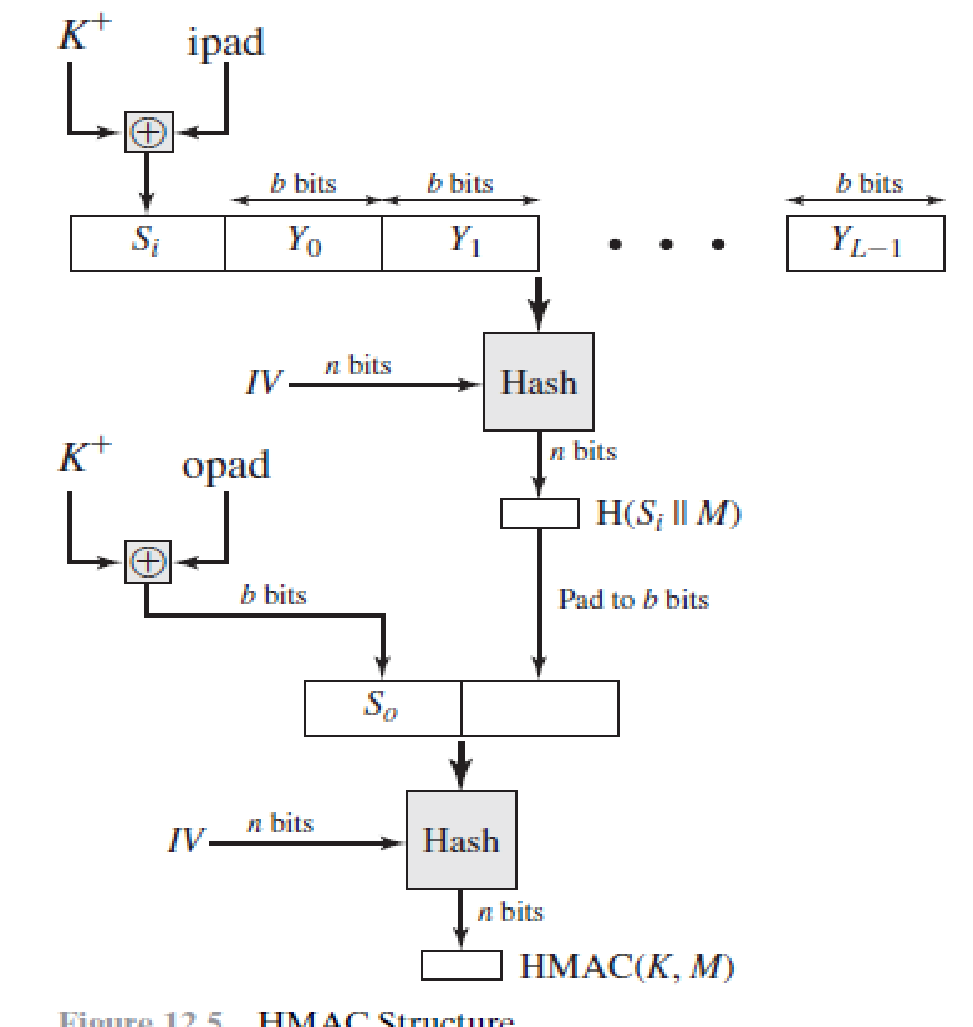n <  K+   <  b

= 36 H = 0011 0110

= 5C H = 0101 1100

HMAC Structure

1. The message is divided into L blocks, each of b bits
2. The secret key K+ is left-padded with 0’s to create a b-bit key. It is recommended that the secret key , before padding be longer than n-bits, where n is the size of the HMAC.
3. The result of step 2 is Exclusive-ORed with a constant called i-pad (input pad) to create a b-bit block. The value of i-pad is the b/8 repetition of the sequence 00110110 (36 in hex).
4. The resulting block is prepended to the L-block  message.
5. The result of step-4 is hashed to create an n-bit digest. We call this as intermediate HMAC.
6. The intermediate HMAC is left-padded with 0’s to make a b-bit block.
7. Step 3 is repeated with a different constant o-pad (output pad). The value of o-pad is the b/8 repetition of the sequence 0101 1100 (5C in hex).
8. The result of step-7 is prepended to the result of step-6.
9. The result of step-8 is hashed with the same hashing algorithm to create the final n-bit HMAC.

Security of HMAC

• Depends in some way on the cryptographic strength of the underlying hash function.
• Appeal of HMAC is that its designers have been able to prove an exact relationship between the strength of the embedded hash function and the strength of HMAC.
• Generally security is expressed in terms of the probability of successful forgery with a given amount of time spent by the forger and a given number of message-tag pairs created with the same key.

Cipher based MAC: DAA and CMAC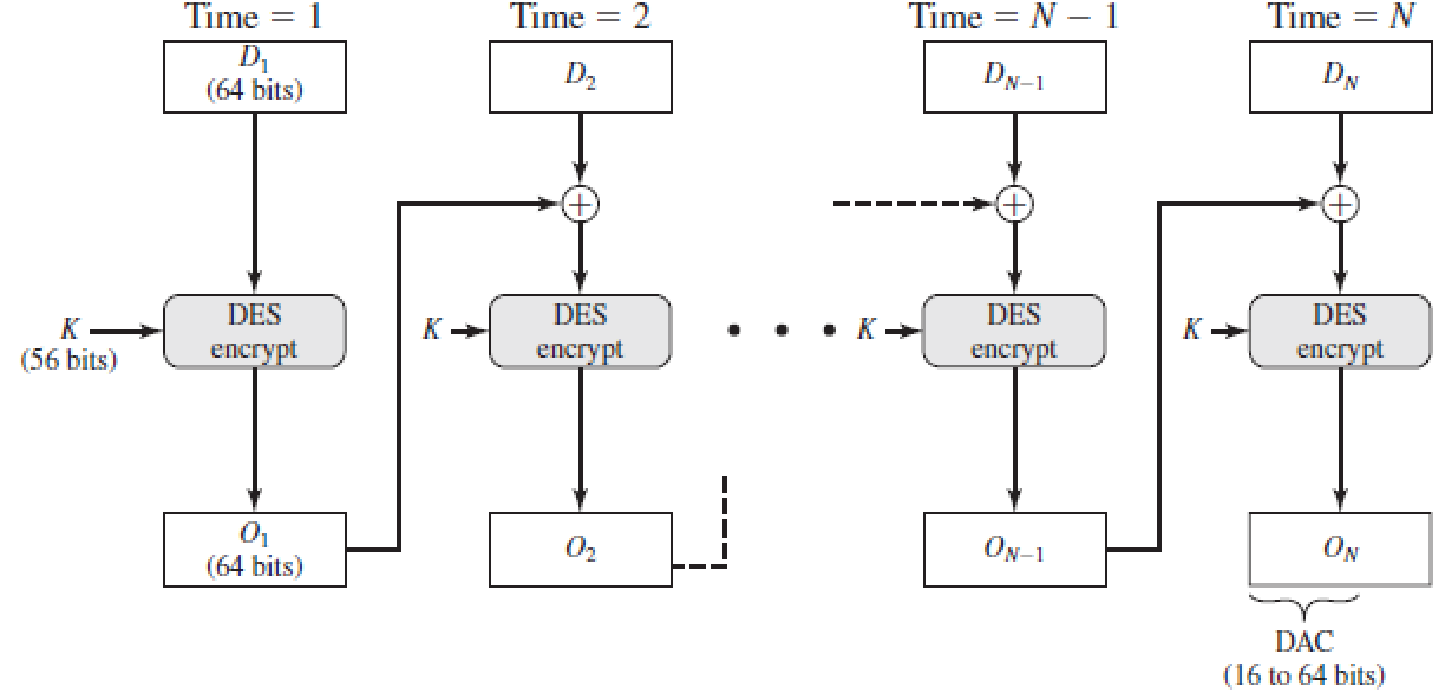• The Data Authentication Algorithm (DAA), is an older algorithm, which is now obsolete.
• It is based on DES, one of the most widely used MACs for a number of years.

The Data Authentication Algorithm  (DAA), based on DES, has been one of the most widely used MACs for a number of years.

However, security weaknesses in this algorithm have been discovered, and it is being replaced by newer and stronger algorithms. The algorithm can be defined as using the cipher block chaining (CBC) mode of operation of DES (Figure 6.4) with an initialization vector of zero. The data (e.g.,message, record, file, or program) to be authenticated are grouped into contiguous

64-bit blocks: D1 , D2 ,. . .  , DN . If necessary, the final block is padded on the right with zeroes to form a full 64-bit block. Using the DES encryption algorithm E and a secret key K , a data authentication code (DAC) is calculated as follows (Figure 12.7).

The Data Authentication Algorithm (DAA)

• DAA use the cipher block chaining (CBC) mode of operation of DES, with an initialization vector of zero. The data to be authenticated are grouped into contiguous 64-bit blocks: D1 , D2 ,. . .  , DN . If necessary, the final block is padded on the right with zeroes to form a full 64-bit block.
• Using the DES encryption algorithm E and a secret key K , a data authentication code (DAC) is calculated as follows

O1 = E(K, D1)

O2 = E(K, [D2 +O1])

O3 = E(K, [D3 + O2])

…………

ON = E(K, [DN+ON-1])

• DAA has been widely adopted in government and industry. This  is secure under a reasonable set of security criteria, with the following restriction
• Only messages of one fixed length of m*n bits are processed, where n is the cipher block size and m is a fixed positive integer.

Cipher-Based Message Authentication Code (CMAC)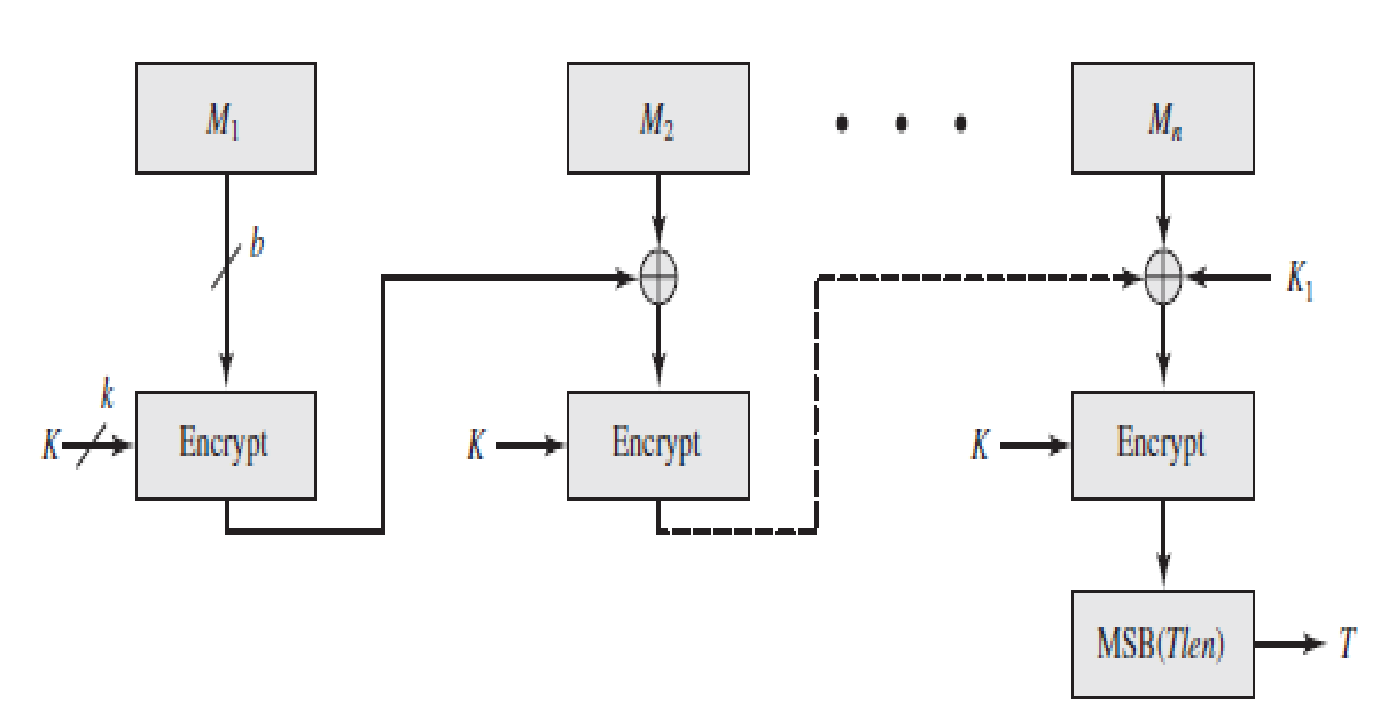• In addition to the symmetric key K of k bits, the CMAC algorithm makes use of another key K1 which is applied only on the last step.
• For AES, the key size k is 128, 192, or 256 bits; for triple DES, the key size is 112 or 168 bits.
• K1 is derived from the encryption algorithm with a plain text of b 0 bits using the cipher key K.
• The n left most bits of last block is the CMAC.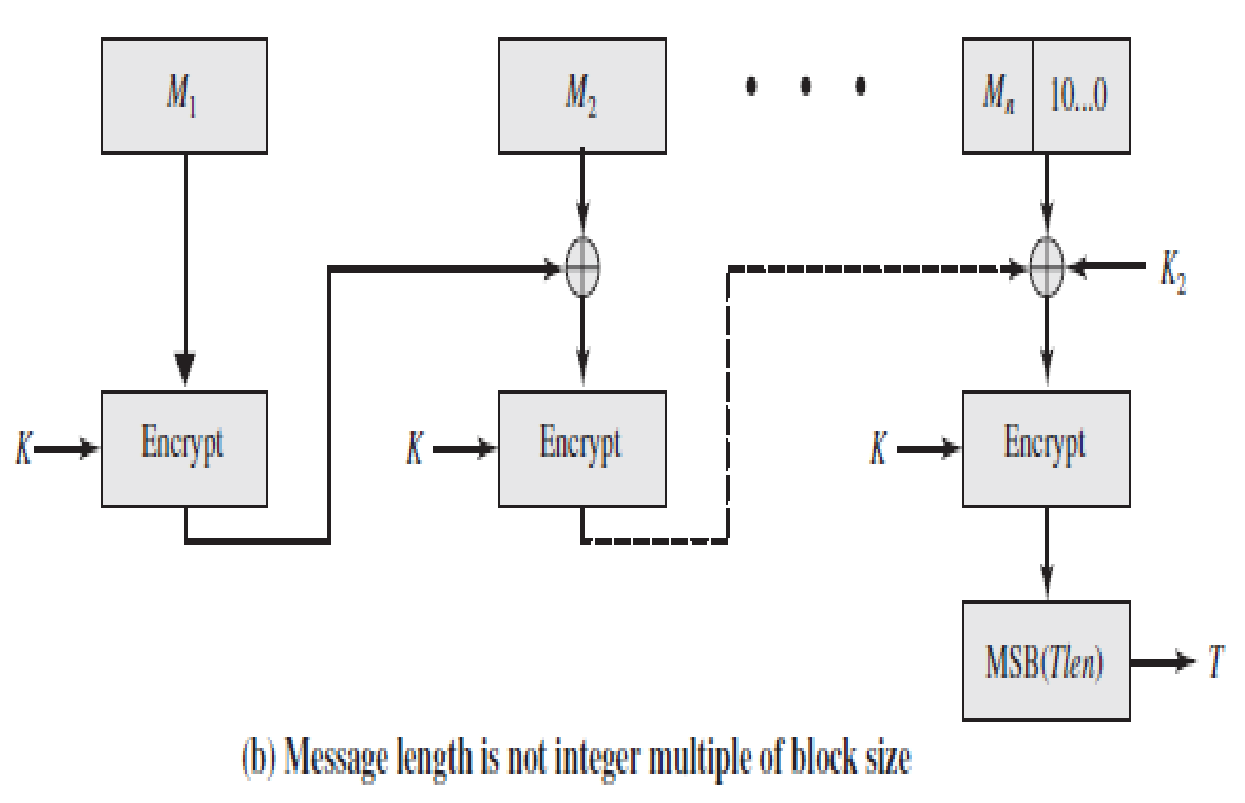• If the message is not an integer multiple of the cipher block length, then the final block is padded to the right (least significant bits) with a 1 and as many 0s as necessary so that the final block is also of length b.
• The CMAC operation then proceeds as before, except that a different b-bit key K2 is used instead of K1.
• The two b-bit keys are derived from the k-bit encryption key as follows.

L = E(K, 0b )

K1 = L . x

K2 = L . x2 = (L . x) . x

• Where x and x2 are first and second- order polynomials that are elements of GF(2b)